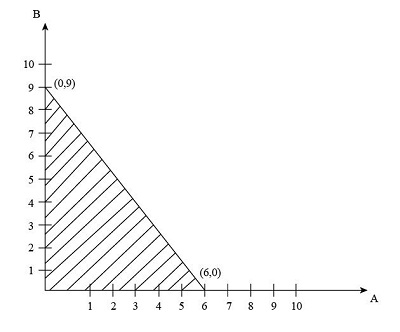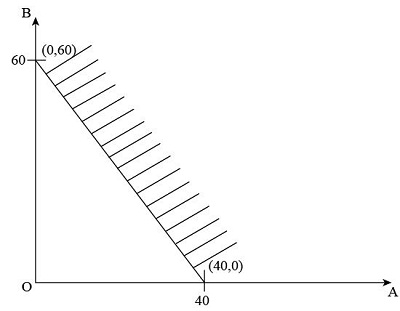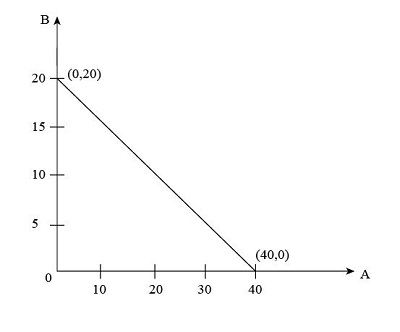# Show a separate graph of the constraint lines and the solutions that satisfy each of the...

## Question:

Show a separate graph of the constraint lines and the solutions that satisfy each of the following constraints:

a. 3A + 2B ? 18

b. 12A + 8B ? 480

c. 5A + 10B = 200

## Cost-Benefit Analyses:

Cost-Benefit Analyses refers to the systematic study of the cost and benefits of any organization, occurred during the business process. Cost-benefit analyses are used by the firms, the government and the various organizations to analyze the impact of any economic activity on the welfare of society.

a. {eq}\begin{array}{l} 3A + 2B \le 18\\ Solution1 = (0,9)\\ Solution2 = (6,0) \end{array} {/eq}In the above graph, the solution of the constraint is represented by the shaded area below the line drawn.

b. {eq}\begin{array}{c} 12A + 8B \ge 480\\ Solution1 = (0,60)\\ Solution1 = (40,0) \end{array} {/eq}In the above graph, the solution of the constraint is represented by the shaded area above the line drawn.

c. {eq}\begin{array}{l} 5A + 10B = 200\\ Solution1 = (0,20)\\ Solution2 = (40,0) \end{array} {/eq}In the above graph, the solution of the constraint is represented by the line drawn.